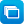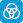Close message Scootle will stop supporting resources that use the Adobe Flash plug-in from 18 Dec 2020. Learning paths that include these resources will have alerts to notify teachers and students that one or more of the resources will be unavailable. Click here for more info.

# Mathematics / Year 7 / Number and Algebra / Linear and non-linear relationships

Curriculum content descriptions

Investigate, interpret and analyse graphs from authentic data (ACMNA180)

Elaborations
• using travel graphs to investigate and compare the distance travelled to and from school
• interpreting features of travel graphs such as the slope of lines and the meaning of horizontal lines
• using graphs of evaporation rates to explore water storage
General capabilities
• Literacy Literacy
• Numeracy Numeracy
• Critical and creative thinking Critical and creative thinking
ScOT terms

Linear equations,  Graphs (Data representation)

## Refine results by

Year level 7-8
Resource type
Learning area Mathematics

## Refine by topic

Related topicExplore the way in which statistics are presented using a wide range of graphs types for ten topics related to human rights. Customise and publish graphs and download data to a spreadsheet program such as Excel to enable further exploration.### A mathematical focus on 'Choose your own statistics'

This teaching resource demonstrates ways in which real data can be interpreted and analysed. It is based on the interactive learning resource Choose your own statistics, in which data is presented as infographics, graphical representations and tables. The focus of the supporting teaching and learning activities is on analysing ...### Filling glasses: graphing and comparing

Look closely at pairs of line graphs. See the relationship between the shape of a glass and the time taken to fill it with juice. Notice that the fluid level rises more quickly in a narrow glass than in a wide glass. Identify the other variables which determine the shape of each line graph. This learning object is one in ...### Filling glasses: create the right graph

Look closely at some line graphs. Examine the relationship between the shape of a glass and the time taken to fill it with juice. Notice that the fluid level rises more quickly in a narrow glass than in a wide glass. Choose both sections of a line graph representing the filling rate for a composite-shaped glass. This learning ...### Trade and Investment at a Glance

Using an illustrated report from the Department of Foreign Affairs and Trade, this Teacher guide provides ten learning sequences that engage students in the analysis and interpretation of data about Australian imports and exports. Students: identify Australia's major exports and imports; investigate international trade ...### Filling glasses: create the right glass

Look closely at some line graphs. Examine the relationship between the shape of a glass and the time taken to fill it with juice. Notice that the fluid level rises more quickly in a narrow glass than in a wide glass. Choose both parts of the composite-shaped glass to match a line graph representing its filling rate. This ...### Filling glasses: find the right glass

Look closely at some line graphs. Examine the relationship between the shape of a glass and the time taken to fill it with juice. Notice that the fluid level rises more quickly in a narrow glass than in a wide glass. Choose the glass shape that matches a line graph representing its filling rate. This learning object is ...### reSolve: Modelling Motion - Year 7

This sequence of seven lessons challenges students to use simple equipment to predict, observe and represent motion. They create a series of graphs to represent motion and construct instruments to measure forces in one and then two dimensions. They interpret these representations to develop concepts of force and motion. ...### Comparative pricing using calculations and linear graphs

This collection of 15 interactive learning objects supports year 7-9 students to use mathematical skills in real life situations involving comparative pricing. Two series, 'Mobile phone plans' and 'School canteen', are used predominantly. Each series offers progressively increasing levels of mathematical application. The ...### Syllabus Bites: Graphing direct proportion

This is the third in a series of Syllabus Bites related to direct and indirect proportion. Students draw graphs to represent relationships between variables in direct proportion. They associate the gradient of the graph with the constant of proportionality. They investigate practical contexts that give rise to direct proportion.### ACMF: Industry facts and figures

This resource is a web page providing data on the Australian chicken meat industry in the form of dynamically generated graphs that allow the user to read the exact figures for any data point. The graphs include chicken meat production in terms of number of birds and tonnage of meat; per capita consumption of chicken, beef, ...### NFF: Farm facts 2012

This is a multilayered web page produced by the National Farmers' Federation, which includes links to a 34-page pdf and a Prezi providing a summary of agricultural production in Australia in 2012. These include information about Australian farmers and farms, as well as an overview of farming sectors such as wool, cotton, ...### Bridge builder: complex squares

Build bridges by adding regular sections (each made up of three beams plus a shared beam). Examine a table and graph of the total number of beams used in bridges of different sizes. Predict the number of beams needed to build a wider span. Describe the number pattern. This learning object is the fourth in a series of five ...### Bridge builder: complex pentagons

Build bridges by adding pentagonal sections (each made up of four beams plus a shared beam). Examine a table and graph of the total number of beams used in bridges of different sizes. Predict the number of beams needed to build a wider span. Describe the number pattern. This learning object is the last in a series of five ...### Solution of simple linear equations

This is a six-page HTML resource about solving problems concerning simple linear equations. It contains five questions, three of which are interactive, and one video. The resource discusses and explains the solution of simple linear equations to reinforce the students' understanding.### Substitute values into simple linear equations

This is a five-page HTML resource about solving problems concerning substitution into simple linear equations. It contains four questions, one of which is interactive, and one video. The resource discusses and explains substitution into simple linear equations to reinforce students' understanding.### Exploring algebra

Calculate a disc jockey's profit or loss when he sells records. Use algebraic notation to represent variables. For example, T = 56 + 3n (where T is total expenses and n is the number of records produced). Calculate revenue and expenses, then work out the profit or loss.### Exploring probability

Look at the probability of a snowboarder choosing any one of six ski runs down a mountain. Compare expected probabilities with experimental results. Examine outcomes illustrated in graphs and probabilities expressed as fractions and percentages. Notice there is likely to be a greater variation between expected and actual ...### Linear Equations

An animated tutorial about solving simple linear equations, followed by an interactive quiz.### Secondary mathematics: using real data

These seven learning activities, which focus on the use of 'real data' using a variety of tools (software) and devices (hardware), illustrate the ways in which content, pedagogy and technology can be successfully and effectively integrated in order to promote learning. In the activities, teachers use the three content strands ...### BBC Bitesize: more on equations - revision

These illustrated information sheets revise the methods of solving linear equations from simple ones with one unknown term to ones with the unknown on both sides. The solution of equations containing brackets or fractions is also considered. Full algebraic working is shown for sample equations. Students solve equations ...### TIMES Module 26: Number and Algebra: linear equations - teacher guide

This is a 16-page guide for teachers. It introduces the solution of linear equations and their uses.